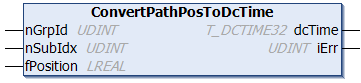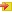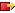# ConvertPathPosToDcTimeThis function block converts a relative Nci path distance to a corresponding 32-bit distributed clock system time variable of type T_DCTIME32 (i.e. the time that corresponds or corresponded to the relative Nci path distance).

##Inputs

`VAR_INPUT    nGrpId    : UDINT;    nSubIdx   : UDINT;    fPosition : LREAL;END_VAR`

Name

Type

Description

nGrpId

UDINT

Group ID of the corresponding Nci channel

nSubIdx

UDINT

This 32-bit input variable contains two different items of information, and is therefore divided into two 16-bit values:

• The LowWord (the least significant 16 bits) contains the subindex for relative addressing of an encoder subelement at an axis. The subindex is counted upwards from zero. For the typical case of an axis that has just one encoder, the null subindex is correct.
• The HighWord (the most significant 16 bits) contains a control word (bit mask) that affects the way in which the position is calculated (e.g. the type of interpolation or extrapolation).
The bit mask 0x0001 means that the set acceleration of the axis is to be included in the calculation.
Bit mask 0x0010 means that the calculation is relative and is currently mandatory. Otherwise the call is rejected with an error.

fPosition

LREAL

Relative Nci path distance converted to the corresponding 32-bit "Distributed Clock System Time".
If the "Distributed Clock System Time" associated with the relative Nci path distance is outside the expected time window of ± 2.147 seconds, this conversion is rejected with an error number.

##Outputs

`VAR_OUTPUT    dcTime : T_DCTIME32;(* 32 bit distributed clock time *)    iErr   : UDINT;END_VAR`

Name

Type

Description

dcTime

T_DCTIME32

Returns the 32-bit "Distributed Clock System Time" variable that corresponds to input fPosition.

iErr

UDINT

Supplies an error number if an error occurs, e.g.

• Error 0x4361: time range exceeded (future)
• Error 0x4362: time range exceeded (past)
• Error 0x4363: position cannot be determined mathematically

## Requirements

Development environment

Target platform

PLC libraries to include

TwinCAT v3.1.0

PC or CX (x86, x64, ARM)

Tc2_EtherCAT